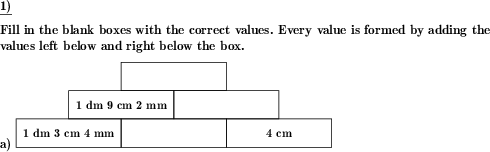Custom math worksheets at your fingertips# Details for problem "Number wall with physical quantities"

Quickname: 5598

Elementary School, Primary School, Junior High School, Middle School.

## Summary

Fill in the blank boxes in the pyramid of units of measurement.

## Example## Description

This is a classic number wall problem with physical units of measurement.

The value for each brick is formed by adding the numbers in the brick left below and right below the brick. Some bricks are left blank - these have to be filled in with the correct value for the unit of measurement.

The number of units that may be covered by a single value can be limited, this means when two units are selected, km dm and m will not be all part of a single specification, but only dm and m, m and dm, dm and cm etc.

Depending on whether both values below an empty box are given, this may be an addition or a subtraction problem.

The wall height is selectable. With a difficulty setting of "easy", it is always the bottom row of the wall that will be given. With a setting of "difficult", other patterns may occur.

Download free printable worksheets for this math problem here. The worksheet contains the problems only, the solution sheet includes the answers. Just click on the respective link.

•Worksheet 1Solution sheet with answers
•Worksheet 2Solution sheet with answers
•Worksheet 3Solution sheet with answers

If you can not see the solution sheets for download, they may be filtered out by an ad blocker that you may have installed. If this is the case, please allow ads for this page and reload the page. The solution sheets will then reappear.

• Do these sample worksheets do not really fit?
• Do you need more math worksheets, with a different level of difficulty?
• Would you like to combine different problems on a worksheet and adjust them to your needs?
• As a teacher, you can put together your own worksheets using the automatically generated math problems provided.
With a free initial credit, you can start creating your own math worksheets in a few minutes.

You can try it for free! Register here, to create custom worksheets now!

## Customization options for this problem

Parameter
Possible values
Number of problems
1, 2, 3, 4, 5
Physical unit
Area (metric), Length (metric), Weight (metric), Time (dhms), Time (hms), Volume litres (metric), Volume dm2 (metric), Money (USD), Money (EUR)
Maximum number of unit levels
2, 3, 4
Height of wall
three, four
Pattern
easy, difficult

## Similar problems

Remark
Description
Similar but with natural numbers
In the number wall missing sums have to be added.
Add and subtract units of measurement
Add and subtract units of measurement